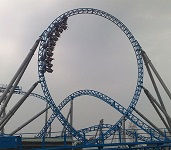# 07: Linear Momentum and Collisions

$$\newcommand{\vecs}{\overset { \rightharpoonup} {\mathbf{#1}} }$$ $$\newcommand{\vecd}{\overset{-\!-\!\rightharpoonup}{\vphantom{a}\smash {#1}}}$$$$\newcommand{\id}{\mathrm{id}}$$ $$\newcommand{\Span}{\mathrm{span}}$$ $$\newcommand{\kernel}{\mathrm{null}\,}$$ $$\newcommand{\range}{\mathrm{range}\,}$$ $$\newcommand{\RealPart}{\mathrm{Re}}$$ $$\newcommand{\ImaginaryPart}{\mathrm{Im}}$$ $$\newcommand{\Argument}{\mathrm{Arg}}$$ $$\newcommand{\norm}{\| #1 \|}$$ $$\newcommand{\inner}{\langle #1, #2 \rangle}$$ $$\newcommand{\Span}{\mathrm{span}}$$ $$\newcommand{\id}{\mathrm{id}}$$ $$\newcommand{\Span}{\mathrm{span}}$$ $$\newcommand{\kernel}{\mathrm{null}\,}$$ $$\newcommand{\range}{\mathrm{range}\,}$$ $$\newcommand{\RealPart}{\mathrm{Re}}$$ $$\newcommand{\ImaginaryPart}{\mathrm{Im}}$$ $$\newcommand{\Argument}{\mathrm{Arg}}$$ $$\newcommand{\norm}{\| #1 \|}$$ $$\newcommand{\inner}{\langle #1, #2 \rangle}$$ $$\newcommand{\Span}{\mathrm{span}}$$$$\newcommand{\AA}{\unicode[.8,0]{x212B}}$$Prince George's Community College General Physics 1030 PHYS 1030 Prof. Neeharika Thakur 7: Momentum & Collisions    8: Static Equilibrium Elasticity & Torque      9: Rotational Kinematics     10: Fluids     11: Fluid Dynamics

• 7.1: Introduction
Linear momentum is the product of the mass and velocity of an object, it is conserved in elastic and inelastic collisions.
• 7.2: Conservation of Momentum
Net external forces (that are nonzero) change the total momentum of the system, while internal forces do not.
• 7.3: Collisions
In an inelastic collision the total kinetic energy after the collision is not equal to the total kinetic energy before the collision.
• 7.4: Rocket Propulsion
In rocket propulsion, matter is forcefully ejected from a system, producing an equal and opposite reaction on what remains.
• 7.5: Center of Mass
The position of COM is mass weighted average of the positions of particles.

Thumbnail: A pool break-off shot. Image used with permission (CC-SA-BY; No-w-ay).

This page titled 07: Linear Momentum and Collisions is shared under a not declared license and was authored, remixed, and/or curated by Boundless.